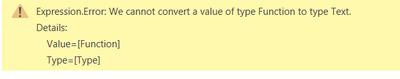cancel
Showing results for
Did you mean:Frequent Visitor

## Using Record.Field to pass column name variable

I'm trying to create a loop in order to create columns from sections in text and seperate the sections out from a big paragraphs.

I first did some test ones that worked perfectly (see the following code)

= Table.AddColumn(#"20.b. Determine Following Section", "LATEX", each Text.BetweenDelimiters([Data],"LATEX",[Next Section after LATEX]))

This outputs exactly what I want.  Change "LATEX" to any of the other items "CAP", "STALK", etc. and they all work how I want them to.

So I decided to put it in a loop.  But apparently I can't just use a variable and pass it through in brackets like so: [Variable]

I read online a lot of people recommend using Record.Field.  But when I use it I constantly get the program reading it as a Function and not text (see image on error).  So I'm not sure what to do.

Here is how I run the function:

= fxSectionInfoGrab(#"20.b. Determine Following Section", Section_Headers)

Here is the function:

let
fxSectionInfoGrab = (DataTable as table, PotentialSectionEnds as table) as any =>
let
// Turn the table into a list
Section_List = Table.ToList(PotentialSectionEnds),

// Determine how many ends are in the list
TotalLoops = List.Count(Section_List),

fxSectionInfo = (DataTableTemp, CurrentLoop) =>
let
// Determine Section Working on
Section_Name = Section_List{CurrentLoop},

// Determine Next Section Column to grab from
Next_Section_Column = "Next Section after " & Section_List{CurrentLoop},

// Determines Next Section Name
//Next_Section = each Record.Field(DataTableTemp, Next_Section_Column),

// Creates TempTable to store
tempTable = Table.AddColumn(DataTableTemp, Section_Name, each Text.BetweenDelimiters([Data], Section_Name, each Record.Field(DataTableTemp, Next_Section_Column)), Text.Type)
in
// Determin the Next Section Name
if CurrentLoop >= TotalLoops-1
then tempTable
else @fxSectionInfo(tempTable, CurrentLoop+1),

//Evaluate the sub-function at the first row
Output = fxSectionInfo(DataTable, 0)
in
Output
in
fxSectionInfoGrabDue to Character requirements I've included a sample of the code in a reply.

1 ACCEPTED SOLUTIONFrequent Visitor

Figured it out on my own.  Here is the code for the function in case anyone was curious.

let
fxSectionInfoGrab = (DataTable as table, PotentialSectionEnds as table) as any =>
let
// Turn the table into a list
Section_List = Table.ToList(PotentialSectionEnds),

// Determine how many ends are in the list
TotalLoops = List.Count(Section_List),

fxSectionInfo = (DataTableTemp, CurrentLoop) =>
let
// Determine Section Working on
Section_Name = Section_List{CurrentLoop},

// Determine Next Section Column to grab from
Next_Section_Column = "Next Section after " & Section_List{CurrentLoop},

// Creates TempTable to store
tempTable = Table.AddColumn(DataTableTemp, Section_Name, each Text.BetweenDelimiters([Data], Section_Name, Record.Field(_, Next_Section_Column)), Text.Type)

in
// Determin the Next Section Name
if CurrentLoop >= TotalLoops-1
then tempTable
else @fxSectionInfo(tempTable, CurrentLoop+1),

//Evaluate the sub-function at the first row
Output = fxSectionInfo(DataTable, 0)
in
Output
in
fxSectionInfoGrab

2 REPLIES 2Frequent Visitor

A sample of the data I'm working with:Frequent Visitor

Figured it out on my own.  Here is the code for the function in case anyone was curious.

let
fxSectionInfoGrab = (DataTable as table, PotentialSectionEnds as table) as any =>
let
// Turn the table into a list
Section_List = Table.ToList(PotentialSectionEnds),

// Determine how many ends are in the list
TotalLoops = List.Count(Section_List),

fxSectionInfo = (DataTableTemp, CurrentLoop) =>
let
// Determine Section Working on
Section_Name = Section_List{CurrentLoop},

// Determine Next Section Column to grab from
Next_Section_Column = "Next Section after " & Section_List{CurrentLoop},

// Creates TempTable to store
tempTable = Table.AddColumn(DataTableTemp, Section_Name, each Text.BetweenDelimiters([Data], Section_Name, Record.Field(_, Next_Section_Column)), Text.Type)

in
// Determin the Next Section Name
if CurrentLoop >= TotalLoops-1
then tempTable
else @fxSectionInfo(tempTable, CurrentLoop+1),

//Evaluate the sub-function at the first row
Output = fxSectionInfo(DataTable, 0)
in
Output
in
fxSectionInfoGrab